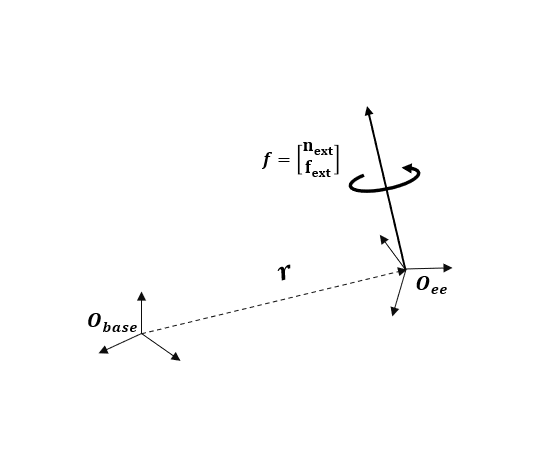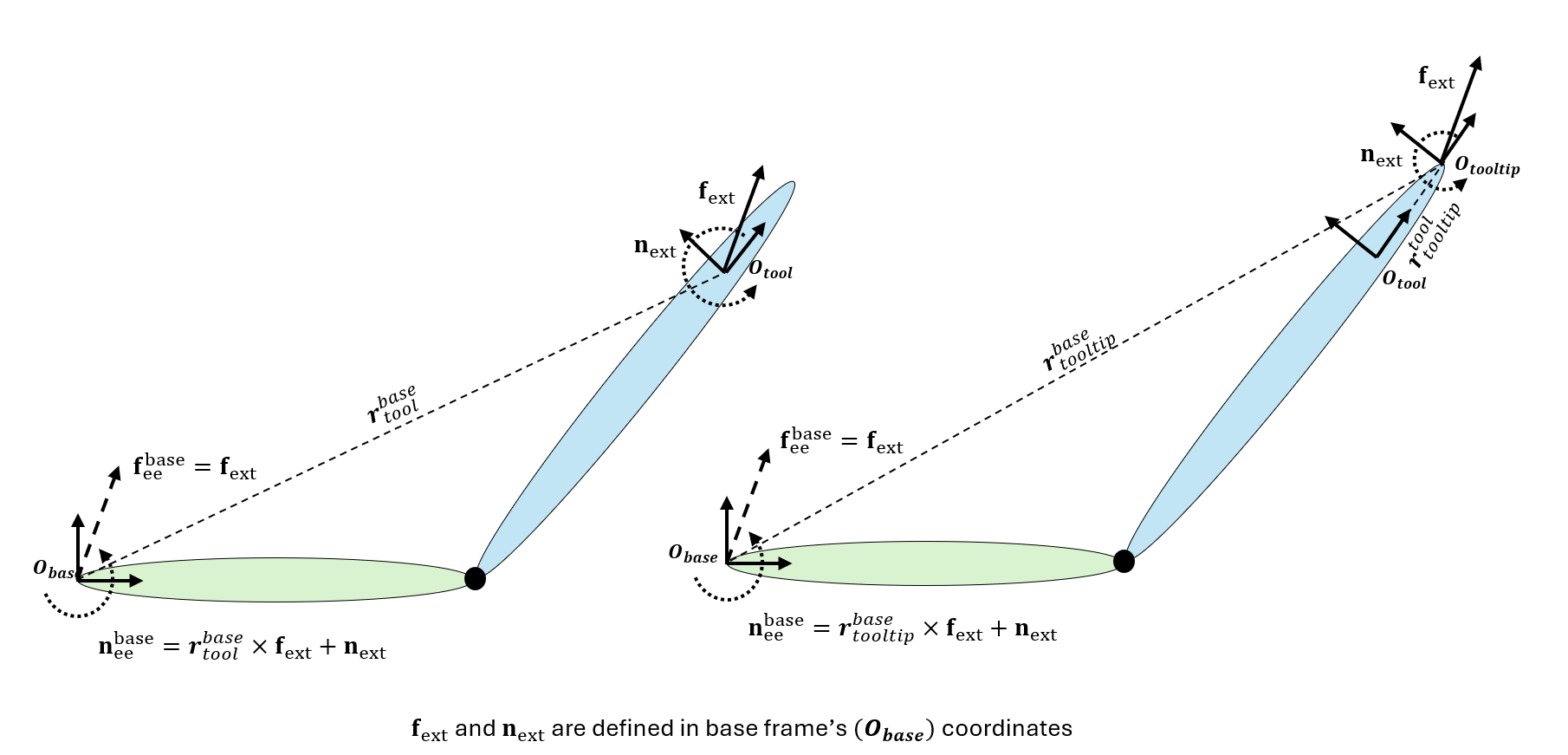# Compute Joint Torques To Balance An Endpoint Force and Moment

Generate torques to balance an endpoint force acting on the end-effector body of a planar robot. To calculate the joint torques using various methods, use the `geometricJacobian`` `and `inverseDynamics` object functions for a `rigidBodyTree` robot model.

### Initialize Robot

The `twoJointRigidBodyTree` robot is a 2-D planar robot. Joint configurations are output as column vectors.

`twoJointRobot = twoJointRigidBodyTree("column");`

### Problem Setup

The endpoint force `eeForce` is a column vector with a combination of the linear force and moment acting on the end-effector body (`"tool"`). Note that this vector is expressed in the base coordinate frame and is shown below.```fx = 2; fy = 2; fz = 0; nx = 0; ny = 0; nz = 3; eeForce = [nx;ny;nz;fx;fy;fz]; eeName = "tool";```

Specify the joint configuration of the robot for the balancing torques.

```q = [pi/3;pi/4]; Tee = getTransform(twoJointRobot,q,eeName);```

### Geometric Jacobian Method

Using the principle of virtual work , find the balancing torque using the `geometricJacobian` object function and multiplying the transpose of the Jacobian by the endpoint force vector.

```J = geometricJacobian(twoJointRobot,q,eeName); jointTorques = J' * eeForce; fprintf("Joint torques using geometric Jacobian (Nm): [%.3g, %.3g]",jointTorques);```
```Joint torques using geometric Jacobian (Nm): [1.41, 1.78] ```

### Inverse Dynamics for Spatially-Transformed Force

Using another method, calculate the balancing torque by computing the inverse dynamics with the endpoint force spatially transformed to the base frame.

Spatially transforming a wrench from the end-effector frame to the base frame means to exert a new wrench in a frame that happens to collocate with the base frame in space, but is still fixed to the end-effector body; this new wrench has the same effect as the original wrench exerted at the ee origin. In the figure below, ${\mathbf{f}}_{\mathbf{ext}}$ and ${\mathbf{n}}_{\mathbf{ext}}\text{\hspace{0.17em}}$ are the endpoint linear force and moment respectively, and the ${\mathbf{f}}_{\mathbf{ee}}^{\mathbf{base}}\text{\hspace{0.17em}}$and ${\mathbf{n}}_{\mathbf{ee}}^{\mathbf{base}\text{\hspace{0.17em}}}$are the spatially transformed forces and moments, respectively. In the snippet below, `fbase_ee` is the spatially transformed wrench.```r = tform2trvec(Tee); fbase_ee = [cross(r,[fx fy fz])' + [nx;ny;nz]; fx;fy;fz]; fext = -externalForce(twoJointRobot, eeName, fbase_ee); jointTorques2 = inverseDynamics(twoJointRobot, q, [], [], fext); fprintf("Joint torques using inverse dynamics (Nm): [%.3g, %.3g]",jointTorques2)```
```Joint torques using inverse dynamics (Nm): [1.41, 1.78] ```

### Inverse Dynamics for End-Effector Force

Instead of spatially transforming the endpoint force to the base frame, use a third method by expressing the end-effector force in its own coordinate frame (`fee_ee`). Transform the moment and the linear force vectors into the end-effector coordinate frame. Then, specify that force and the current configuration to the `externalForce` function. Calculate the inverse dynamics from this force vector.

```eeLinearForce = Tee \ [fx;fy;fz;0]; eeMoment = Tee \ [nx;ny;nz;0]; fee_ee = [eeMoment(1:3); eeLinearForce(1:3)]; fext = -externalForce(twoJointRobot,eeName,fee_ee,q); jointTorques3 = inverseDynamics(twoJointRobot, q, [], [], fext); fprintf("Joint torques using inverse dynamics (Nm): [%.3g, %.3g]",jointTorques3);```
```Joint torques using inverse dynamics (Nm): [1.41, 1.78] ```

### References

Siciliano, B., Sciavicco, L., Villani, L., & Oriolo, G. (2009). Differential kinematics and statics. Robotics: Modelling, Planning and Control, 105-160.

Harry Asada, and John Leonard. 2.12 Introduction to Robotics. Fall 2005. Chapter 6 Massachusetts Institute of Technology: MIT OpenCourseWare, https://ocw.mit.edu. License: Creative Commons BY-NC-SA.